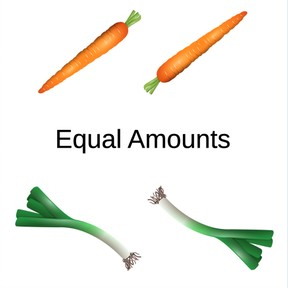Recognizing equal quantities to 5

# Recognizing equal quantities to 5

Recognizing equal quantities to 58,000 schools use Gynzy92,000 teachers use Gynzy1,600,000 students use Gynzy

## General

Students learn to recognize quantities that are equal to 5. They learn this by comparing different quantities to each other.

K.CC.C.6
K.CC.C.7

## Introduction

Ask students to form pairs and practice recognizing quantities to 5. The first student holds up a few fingers on one hand, the second student must name the quantity. They take turns. Next practice with counting to 5 by counting all of the different fruits.

## Instruction

Choose two students to come to the front of the class and give each student 4 books. Explain the word equal to them. How many books does the first student have? How many does the other have? Both students have 4 books so that is an equal quantity. How do you know? First count one group, and then the other group. If the numbers are the same, they have equal quantities. Practice this with an unequal set to demonstrate the difference.

Check that students know what equal quantities are with a game. The students form pairs and get 5 blocks each. By turns one student puts down a number of blocks, and the other must put down an equal quantity. Finally, practice with the dice to compare with the images.

To check that students understand what equal quantities are the teacher can ask the following questions:
- What does 'equal quantities' mean?
- How do you know if there are equal quantities?

## Guided Practice

The students practice by comparing different images with quantities to 5 and seeing if they are equal quantities or not.

## Closing

Check that students can recognize equal quantities up to 5. Ask how they figured out their answer. Do you see it right away? Or do you have to count? Close with a game where students put down as many blocks as fruit are displayed on the interactive whiteboard.

## Teaching Tip

Students who struggle should be encouraged to practice counting 1-5. Students ready to be challenged can already practice with quantities to 10 or practice with numbers rather than images.

## Instruction materials

Books, blocks, dice

### The online teaching platform for interactive whiteboards and displays in schools

• Save time building lessons

• Manage the classroom more efficiently

• Increase student engagement